## Expanding Cubed Binomials: Algebra 2/Trig.

Greetings math friends! This post will go over expanding cubed binomials using two different methods to get the same answer. We’re so used to seeing squared binomials such as,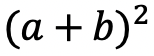, and expanding them without a second thought.  But what happens when our reliable squared binomials are now raised to the third power, such as,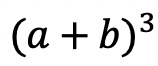?  Luckily for us, there is a Rule we can use:

But where did this rule come from?  And how can we so blindly trust it? In this post we will prove why the above rule works for expanding cubed binomials using 2 different methods:

Why bother? Proving this rule will allow us to expand and simplify any cubic binomial given to us in the future! And since we are proving it 2 different ways, you can choose the method that best works for you.

## Method #1: The Box Method

Step 1: First, focus on the left side of the equation by expanding (a+b)3:

Step 2: Now we are going to create our first box, multiplying (a+b)(a+b). Notice we put each term of (a+b) on either side of the box. Then multiplied each term where they meet.

Step 3: Combine like terms ab and ab, then add each term together to get a2+2ab+b2.

Step 4: Multiply (a2+2ab+b2)(a+b) making a bigger box to include each term.

Step 5: Now combine like terms (2a2b and a2b) and (2ab2 and ab2), then add each term together and get our answer: a3+3a2b+3ab2+b3.

## Method #2: The Distribution Method

Let’s expand the cubed binomial using the distribution method step by step below:

Now that we’ve gone over 2 different methods of cubic binomial expansion, try the following practice questions on your own using your favorite method!

Practice Questions: Expand and simplify the following.

Solutions:

Still, got questions?  No problem! Check out the video above or comment below! Happy calculating! 🙂

**Bonus: Test your skills with this Regents question on!

## Piecewise Functions: Algebra

Greetings, today’s post is for those in need of a piecewise functions review!  This will cover how to graph each part of that oh so intimidating piecewise functions.  There’s x’s, there are commas, there are inequalities, oh my! We’ll figure out what’s going on here and graph each part of the piecewise-function one step at a time.  Then check yourself with the practice questions at the end of this post. Happy calculating! 🙂

## What are Piece-Wise Functions?

Exactly what they sound like! A function that has multiple pieces or parts of a function.  Notice our function below has different pieces/parts to it.  There are different lines within, each with their own domain.

Now let’s look again at how to solve our example, solving step by step:

Translation: We are going to graph the line f(x)=x+1 for the domain where x > 0

To make sure all our x-values are greater than or equal to zero, we create a table plugging in x-values greater than or equal to zero into the first part of our function, x+1.  Then plot the coordinate points x and y on our graph.

Translation: We are going to graph the line  f(x)=x-3 for the domain where x < 0.

To make sure all our x-values are less than zero, let’s create a table plugging in negative x-values values leading up to zero into the second part of our function, x-3.  Then plot the coordinate points x and y on our graph.

## Practice Questions:

Graph each piecewise function:

## Solutions:

Still got questions?  No problem! Check out the video above or comment below for any questions. Happy calculating! 🙂

***Bonus! Want to test yourself with a similar NYS Regents question on piecewise functions?  Click here.

## Intersecting Secants Theorem: Geometry

Ahoy! Today we’re going to cover the Intersecting Secants Theorem!  If you forgot what a secant is in the first place, don’t worry because all it is a line that goes through a circle.  Not so scary right? I was never scared of lines that go through circles before, no reason to start now.

If you have any questions about anything here, don’t hesitate to comment below and check out my video for more of an explanation. Stay positive math peeps and happy calculating! 🙂

Wait, what are Secants?

Intersecting Secants Theorem: When secants intersect an amazing thing happens! Their line segments are in proportion, meaning we can use something called the Intersecting Secants Theorem to find missing line segments.  Check it out below:

Let’s now see how we can apply the intersecting Secants Theorem to find missing length.

Step 1: First, let’s write our formula for Intersecting Secants.

Step 2: Now fill in our formulas with the given values and simplify.

Step 3: All we have to do now is solve for x! I use the product.sum method here, but choose the factoring method that best works for you!

Step 4: Since we have to reject one of our answers, that leaves us with our one and only solution x=2.

Practice Questions: Find the value of the missing line segments x.

Solutions:

Still got questions?  No problem! Check out the video above or comment below for any questions and follow for the latest MathSux posts. Happy calculating! 🙂

To review a similar NYS Regents question check out this post here.

## Circle Theorems & Formulas

Inscribed Angles & Intercepted Arcs

Area of a Sector

Circle Theorems

## How to Graph Equation of a Line, y=mx+b: Algebra

Hi everyone, welcome back to Mathsux! This week we’ll be reviewing how to graph an equation of a line in y=mx+b form. And if you have not checked out the video below, please do! Happy calculating! 🙂

An equation of a line can be represented by the following formula:

y=mx+b

Y-Intercept: This is represented by b, the stand-alone number in y=mx+b. This represents where the line hits the y-axis.  This is always the first point you want to start with when graphing at coordinate point (0,b).

Slope: This is represented by m, the number next to x in y=mx+b. Slope tells us how much we go up or down the y-axis and left or right on the x- axis in fraction form:

Now let’s check out an Example!

Graph the following: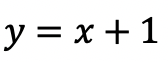-> First, let’s identify the slope and y-intercept of our line.

-> To start, let’s graph the first point on our graph, the y-intercept at point (0,1):

-> Now for the slope. We are going to go up one and over to the right one for each point, since our slope is 1/1.

-> Connect all of our coordinate points and label our graph.

Try the following practice questions on your own!

Practice Questions:

Want more Mathsux?  Don’t forget to check out our Youtube channel and more below! And if you have any questions, please don’t hesitate to comment below. Happy Calculating! 🙂

Need to brush up on slope? Click here to see how to find the rate of change.

## 4 Ways to Factor Trinomials: Algebra

Greeting math peeps and welcome to MathSux! In this post, we are going to go over 4 ways to Factor Trinomials and get the same answer, including, (1) Quadratic Formula (2) Product/Sum, (3) Completing the Square, and (4) Graphing on a Calculator.  If you’re looking for more don’t forget to check out the video and practice questions below.  Happy Calculating! 🙂

Also, if need a review on Factor by Grouping or Difference of Two Squares (DOTS) check out the hyperlinks here!

*If you haven’t done so, check out the video that goes over this exact problem, and don’t forget to subscribe!

We will take this step by step, showing 4 ways to factor trinomials, getting the same answer each and every time! Let’s get to it!

## 4 Ways to Factor Trinomials

____________________________________________________________________

## (2) Product/Sum: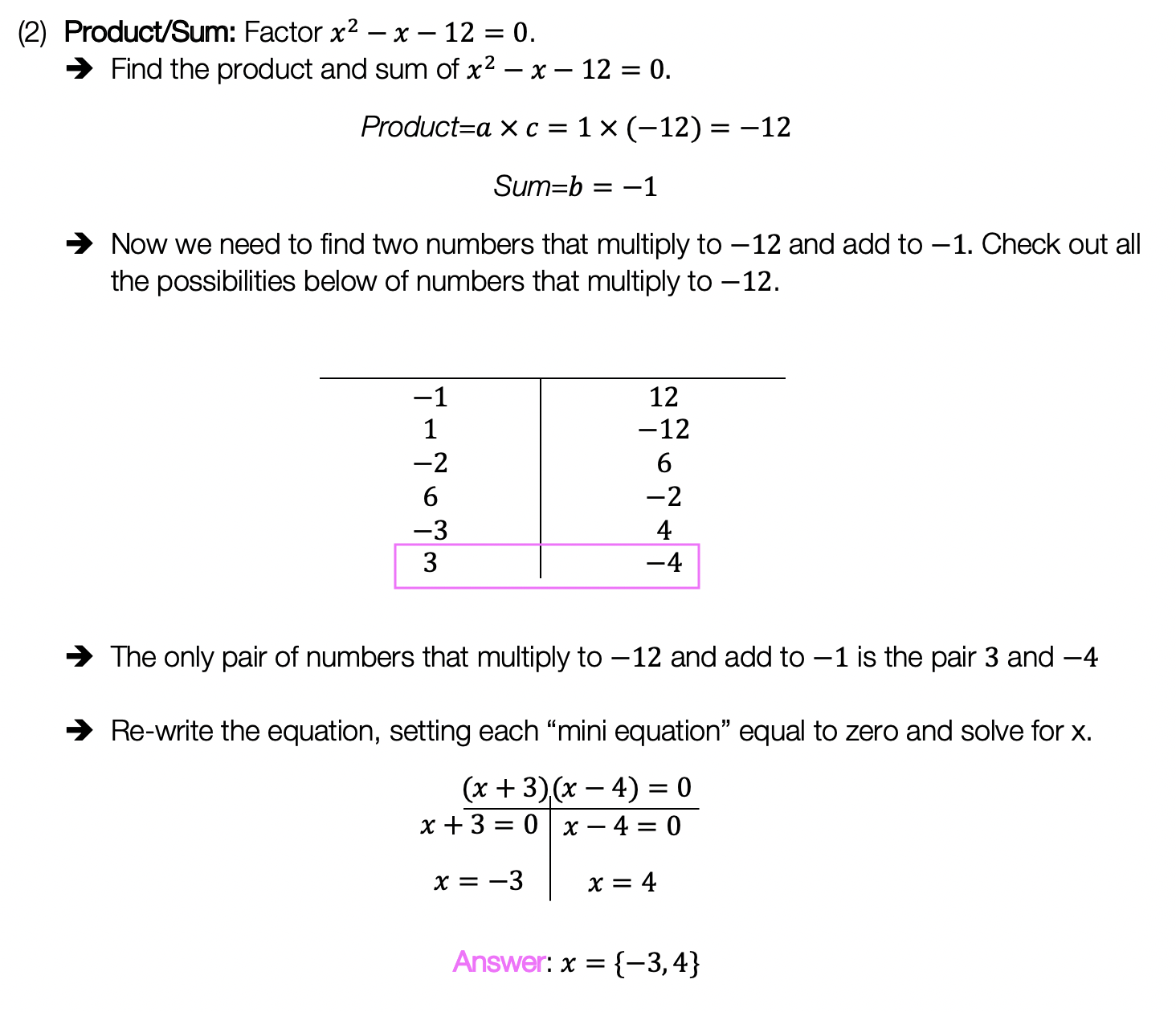____________________________________________________________________

## (3) Completing the Square: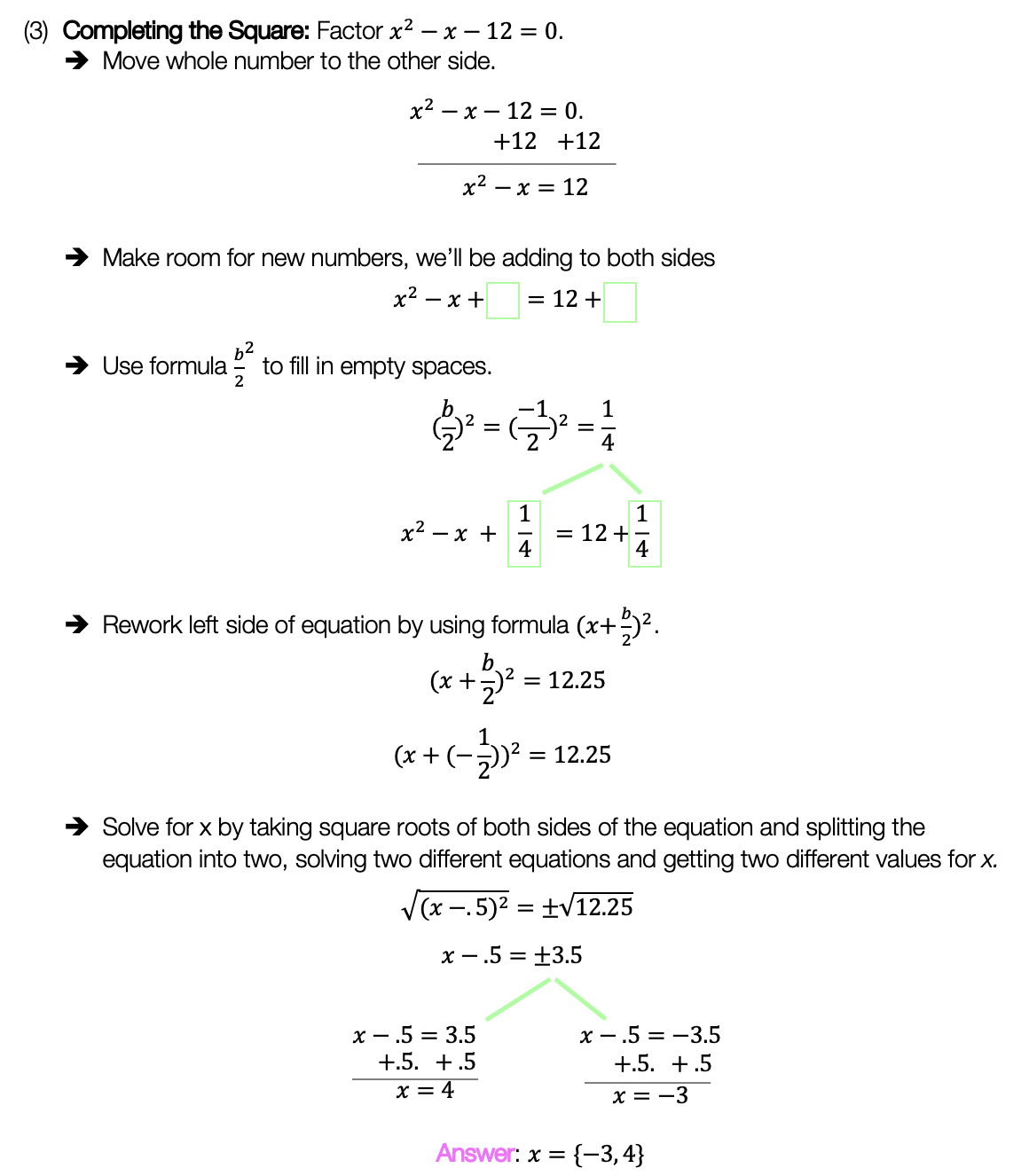____________________________________________________________________

## (4) Graph:

Choose the factoring method that works best for you and try the practice problems on your own below!

Practice Questions:

Solutions:

Want a review of all the different factoring methods out there?  Check out the ones left out here (DOTS and GCF) and happy calculating! 🙂

For even more ways to factor quadratic equations, check out How to factor by Grouping here! 🙂

Looking for more on Quadratic Equations and functions? Check out the following Related posts!

Factoring Review

Factor by Grouping

Completing the Square

The Discriminant

Is it a Function?

Imaginary and Complex Numbers

Quadratic Equations with 2 Imaginary Solutions

Focus and Directrix of a Parabola

Also, if you want more Mathsux?  Don’t forget to check out our Youtube channel and more below! If you have any questions, please don’t hesitate to comment below. Happy Calculating! 🙂

## Median of a Trapezoid Theorem: Geometry

Hi everyone and welcome to Math Sux! In this post, we are going to look at how to use and applythe median of a trapezoid theorem. Thankfully, it is not a scary formula, and one we can easily master with a dose of algebra. The only hard part remaining, is remembering this thing! Take a look below to see a step by step tutorial on how to use the median of a trapezoid theorem and check out the practice questions at the end of this post to truly master the topic. Happy calculating! 🙂

*If you haven’t done so, check out the video that goes over this exact problem, also please don’t forget to subscribe!

Step 1:  Let’s apply the Median of a Trapezoid Theorem to this question!  A little rusty?  No problem, check out the Theorem below.

Median of a Trapezoid Theorem: The median of a trapezoid is equal to the sum of both bases.Step 2: Now that we found the value of x , we can plug it back into the equation for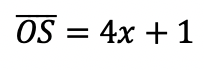median,  to find the value of median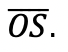Want more practice?  Your wish is my command! Check out the practice problems below:

Practice Questions:

1.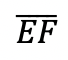is the median of trapezoid ABCDEF, find the value of the median, given the following:2.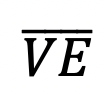is the median of trapezoid ACTIVE, find the value of the median, given the following:3.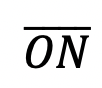is the median of  trapezoid DRAGON, find the value of the median, given the following:

4.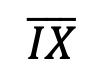is the median of trapezoid MATRIX, find the value of the median, given the following:

Solutions:

Need more of an explanation?  Check out the detailedand practice problems. Happy calculating! 🙂

## Completing the Square: Algebra

Want to learn the ins and out of completing the square?  Then you’ve come to the right place! Learn how to Complete the Square step by step in the video and article below, then try the practice problems at the end of this post to truly master the topic! If you’re looking for more on completing the square, check out this post here. Happy Calculating! 🙂

Check out the video below for an in-depth look at completing the square: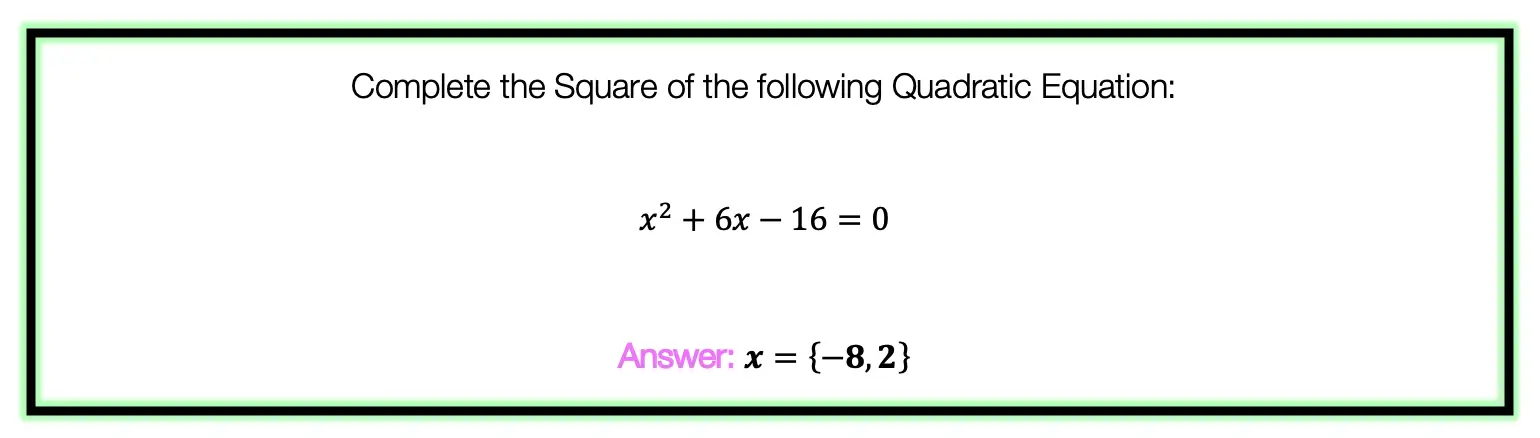To answer this question, there are several steps we must follow including:

Step 1: Move the whole number, which in this case is 16, to the other side of the equation.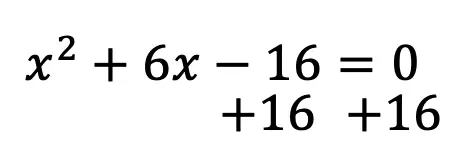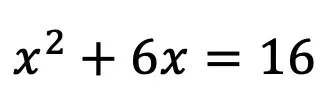Step 2: Make space for our new number on both sides of the equation.  This number is going to be found by using a particular formula shown below: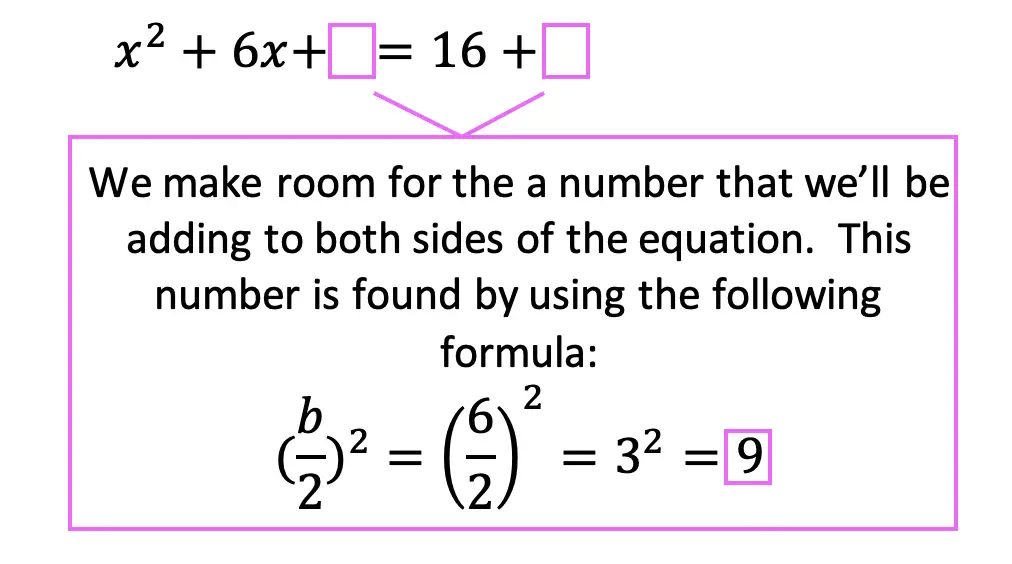Step 3: Add the number 9 to both sides of the equation, which we found using our formula.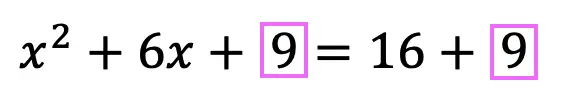Step 4: Combine like terms on the right side of the equation, adding 16+9 to get 25.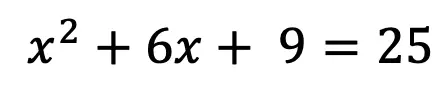Step 5: Now, we need to re-write the left side of the equation using the following formula.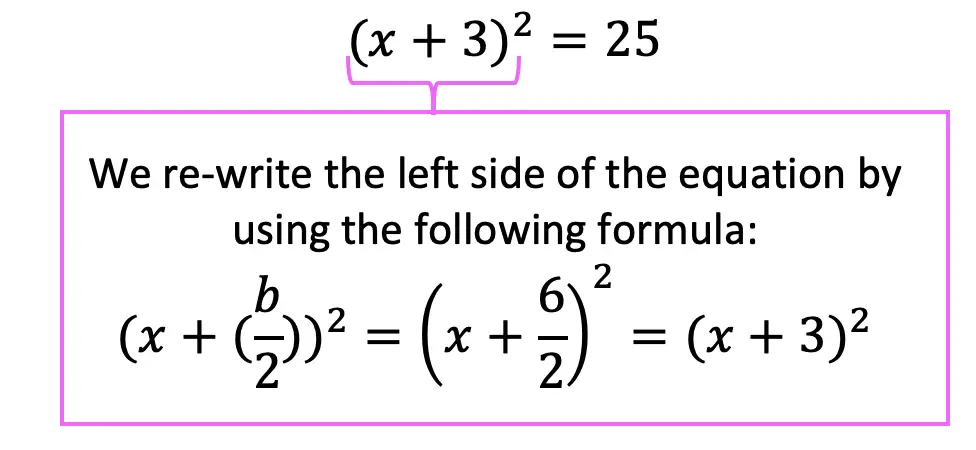Step 6: Finally, we solve for x by taking the positive and negative square root to get the following answer and solve for two different equations: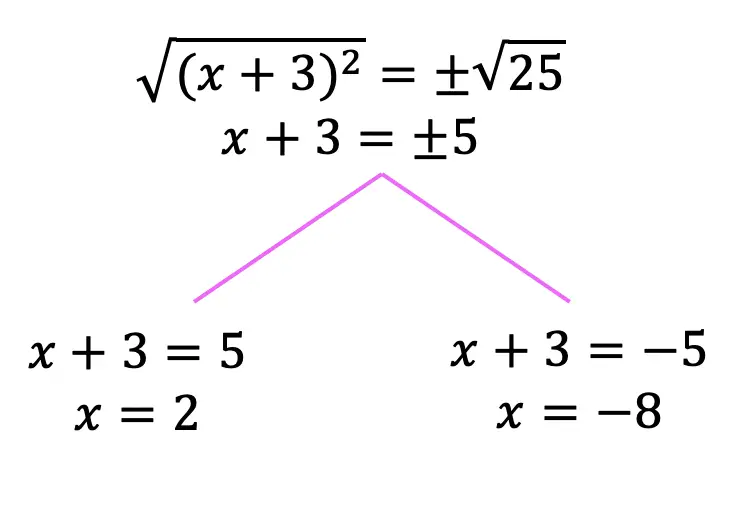Practice Questions: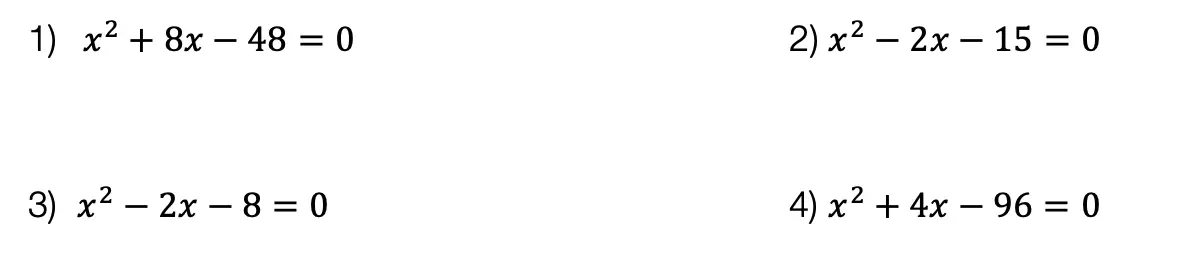Solutions: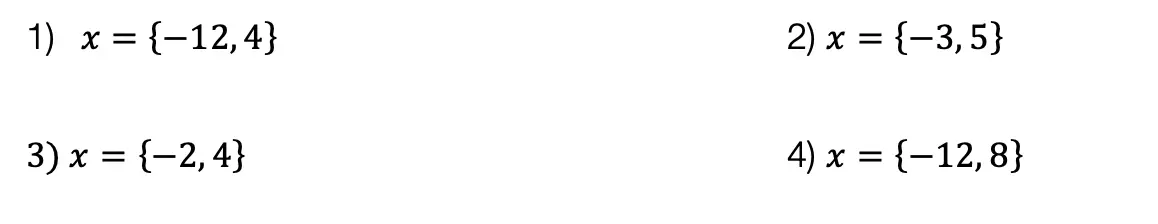Want more Mathsux?  Don’t forget to check out our Youtube channel and more below! And if you have any questions, please don’t hesitate to comment below. Happy Calculating! 🙂

Need more of an explanation?  Check out why we complete the square in the first place here ! 🙂

Looking for more on Quadratic Equations and Functions? Check out the following Related posts!

Factoring Review

Factor by Grouping

Is it a Function?

The Discriminant

4 Ways to Factor Trinomials

Imaginary and Complex Numbers

Quadratic Equations with 2 Imaginary Solutions

Focus and Directrix of a Parabola

## Solving Radical Equations: Algebra 2/Trig.

Today we’re back with Algebra 2, this time solving for radical equations!  Did you say “Radical Equations?” As in wild and crazy equations? No, not exactly, radicals in math are used to take the square root, cubed root, or whatever root of a number.

## Example #1:

Radicals are actually pretty cool because we can write them a couple of different ways and they all mean the same thing! Check it out below:Still not sure of their coolness? Let’s see what they look like with actual numbers:
Example: Solve the following algebraic equation below for the missing variable (aka, solve for x).Explanation:

How do I answer this question?

The question wants us to solve for x using our knowledge of radicals and algebra. You can also check out how to solve this question on Youtube here!

How do we do this?

Step 1: We start solving this radical equation like any other algebraic problem: by getting x alone. We can do this easily by subtracting 7 and then dividing out 5.

Step 2: Now, to get rid of that pesky radical, we need to square the entire radical.  Remember, whatever we do to one side of the equation, we must also do to the other side of the equation, therefore, we also square 14 on the other side of the equal sign. *This gets rid of our radical and allows us to solve for x algebraically as normal!

What happens when there is a cubed root though!?!?

When dividing polynomials with different value roots, raise the entire radical to that same power of root to cancel it out:Remember, we know radicals can also be written as fractions:

Therefore we also know that if we raise the entire radical expression to the same power of the root, the two exponents will cancel each other out:

## Example #2:

Want more practice? Try solving radical equations in the next few examples on your own.

## Solutions:

Looking to brush up on how to solve absolute value equations? Check out the post here! Did I miss anything?  Don’t let any questions go unchecked and let me know in the comments! Happy calculating! 🙂

Don’t forget to check out the latest free videos and posts with MathSux and subscribe!

## Earth Day Fractals!

In honor of Earth Day last week, I thought we’d take a look at some math that appears magically in nature.  Math? In nature?  For those of you who think math is unnatural or just terrible in general, this is a great time to be proven otherwise!

The key that links math to nature is all about PATTERNS. All math is based on is patterns.  This includes all types of math, from sequences to finding x, each mathematical procedure follows some type of pattern. Meanwhile back in the nearest forest, patterns are occurring everywhere in nature.

The rock star of all patterns would have to be FRACTALS. A Fractal is a repeating pattern that is ongoing and has different sizes of the exact same thing!  And the amazing thing is that we can actually find fractals in our neighbor’s local garden.

Let’s look at some Fractal Examples:

(1) Romanesco Broccoli:  Check out those repeating shapes, that have the same repeating shapes on those shapes and the same repeating shapes on even smaller shapes and…. my brain hurts!

######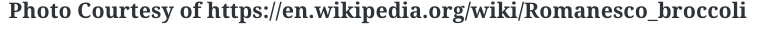(2) Fern Leaves:  The largest part of this fractal is the entire fern leaf itself.  The next smaller and identical part is each individual “leaf” along the stem.  If you look closely, the pattern continues!

######(3) Leaves:  If you’ve ever gotten up real close to any type of leaf, you may have noticed the forever repeating pattern that gets smaller and smaller. Behold the power and fractal pattern of this mighty leaf below!

######.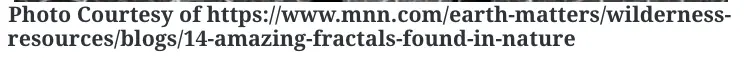Just in case fractals are still a bit hard to grasp, check out the most famous Fractal below,  otherwise known as Sierpinski’s Triangle.  This example might not be found in your local back yard, but it’s the best way to see what a fractal truly is up close and infinite and stuff.

Looking for more math in nature?  Check out this post on the Golden Ratio and happy calculating! 🙂

|    |